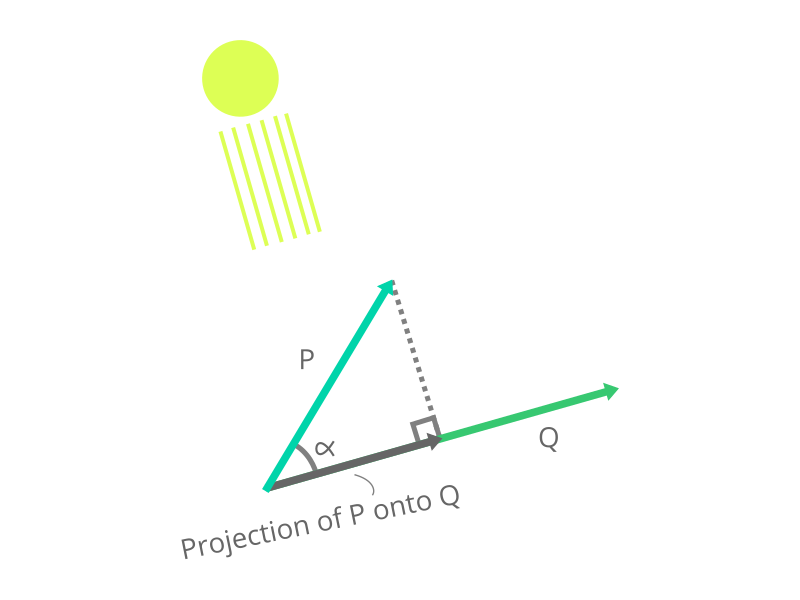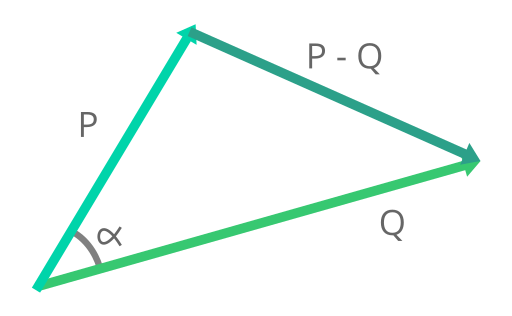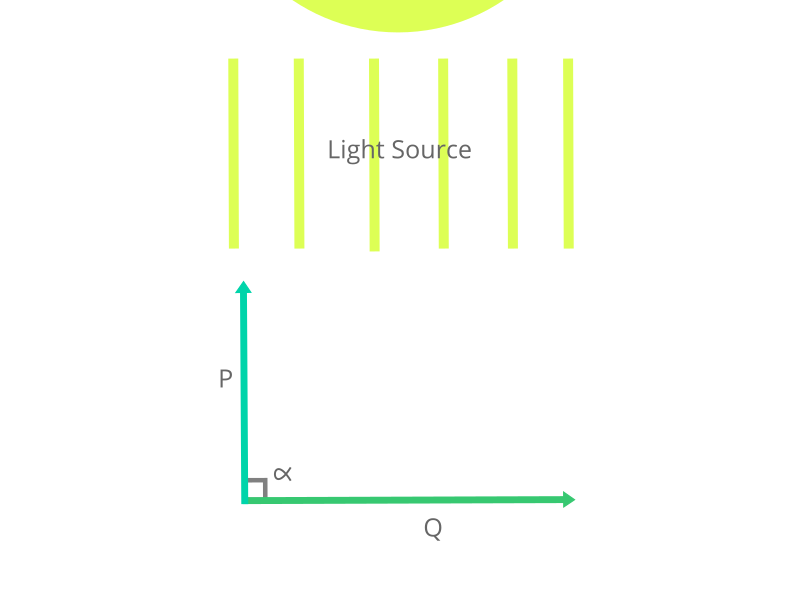# Why is the Dot Product of Two Perpendicular Vectors Zero?

#### Posted by Sean Francis N. Ballais on May 3, 2020 06:38 PM

Since last week, I have been teaching myself some linear algebra through Eric Lengyel’s’ book, Mathematics for 3D Game Programming and Computer Graphics. One of the topics I have recently learned is about the dot product of vectors. The dot product is simply the length of the projection of one vector onto the other vector. You could think of a projection as a shadow of the vector being projected, on the other vector where the light source is perpendicular and is pointing to the other vector. The picture below shows a visualization of what I just described. In the image below, $$P$$ and $$Q$$ are 2D vectors. Note that the projection is also another vector.The dot product is defined as:

$P \cdot Q = \sum_{i=1}^{n} P_{i}Q_{i}$

where $$P$$ and $$Q$$ are $$n$$-dimensional vectors. From the definition alone, we can see that the dot product is just a summation of the products of each component from each vector. For example, let’s say we have two 2D vectors, $$P = \langle 2, 3 \rangle$$ and $$Q = \langle 4, 2 \rangle$$. The dot product of those two vectors would go as follows.

\begin{align} P \cdot Q & = \sum_{i=1}^{n} P_{i}Q_{i} \\ & = P_{1}Q_{1} + P_{2}Q_{2} \\ & = (2)(4) + (3)(2) \\ & = 8 + 6 \\ P \cdot Q & = 14 \end{align}

One property that we can obtain from the dot product is that, whenever we get the dot product of two perpendicular vectors, the result is zero. Why is it so? We can use the basic definition of the dot product to answer that, but using another definition will provide us with another perspective that will give us a slightly more in-depth understanding why it is so. But, what is that other definition?

Before I directly answer that, let’s try something else first. Let’s take two vectors again, $$P$$ and $$Q$$. Let’s make a vector that starts from the head of $$P$$ and ends at the head of $$Q$$. Noticeably, we get a vector that is just $$P - Q$$, and a triangle made from the three vectors.Now, let’s play with the length, also known as the magnitude of the vector $$P - Q$$. Remember that the magnitude of a vector $$P$$ is symbolized as $$\left | \left | P \right |\right |$$, and is defined by the equation below. Some texts use $$| P |$$ for the magnitude. I do not recommend using that notation because it might be confused with the absolute value. Also, looking closely at the equation below, you will notice that the magnitude definition is based off of the Pythagorean theorem.

$\left \| P \right \| = \sqrt{\sum_{i=1}^{n} P_{i}^{2}}$

Interestingly, the above is not the only equation for the length of $$P - Q$$. Since we got a triangle, we can actually utilize trigonometry to get the length. The Law of Cosines allows us to obtain another equation for the length. Utilizing said law would give us the equation.

$\left \| P - Q \right \| = \sqrt{\left \| P \right \|^{2} + \left \| Q \right \|^{2} - 2\left \| P \right \|\left \| Q \right \|\cos(\alpha)}$

$$\alpha$$ in the equation is simply the angle between vectors, $$P$$ and $$Q$$. To make the equation easier to play with, let’s remove the square root from the equation.

$\left \| P - Q \right \|^{2} = \left \| P \right \|^{2} + \left \| Q \right \|^{2} - 2\left \| P \right \|\left \| Q \right \|\cos(\alpha)$

Let’s play around with the equation further.

\begin{align} \left \| P - Q \right \|^{2} & = \left \| P \right \|^{2} + \left \| Q \right \|^{2} - 2\left \| P \right \|\left \| Q \right \|\cos(\alpha) \\ \left (\sqrt{\sum_{i=1}^{n}(P_{i} - Q_{i})^{2}} \; \right )^{2} & = \left (\sqrt{\sum_{i=1}^{n}P_{i}^{2}} \; \right )^{2} + \left (\sqrt{\sum_{i=1}^{n}Q_{i}^{2}} \; \right)^{2} - 2\left \| P \right \|\left \| Q \right \|\cos(\alpha) \\ \sum_{i=1}^{n}(P_{i} - Q_{i})^{2} & = \sum_{i=1}^{n}P_{i}^{2} + \sum_{i=1}^{n}Q_{i}^{2} - 2\left \| P \right \|\left \| Q \right \|\cos(\alpha) \\ & = \sum_{i=1}^{n}(P_{i}^{2} + Q_{i}^{2}) - 2\left \| P \right \|\left \| Q \right \|\cos(\alpha) \\ \sum_{i=1}^{n}(P_{i}^{2} - 2P_{i}Q_{i} + Q_{i}^{2}) & = \sum_{i=1}^{n}(P_{i}^{2} + Q_{i}^{2}) - 2\left \| P \right \|\left \| Q \right \|\cos(\alpha) \\ \sum_{i=1}^{n}(P_{i}^{2} - 2P_{i}Q_{i} + Q_{i}^{2}) - \sum_{i=1}^{n}(P_{i}^{2} + Q_{i}^{2}) & = -2\left \| P \right \|\left \| Q \right \|\cos(\alpha) \\ \sum_{i=1}^{n}(P_{i}^{2} - 2P_{i}Q_{i} + Q_{i}^{2}) - (P_{i}^{2} + Q_{i}^{2}) & = -2\left \| P \right \|\left \| Q \right \|\cos(\alpha) \\ \sum_{i=1}^{n}(P_{i}^{2} - 2P_{i}Q_{i} + Q_{i}^{2} - P_{i}^{2} - Q_{i}^{2}) & = -2\left \| P \right \|\left \| Q \right \|\cos(\alpha) \\ \sum_{i=1}^{n}(-2P_{i}Q_{i}) & = -2\left \| P \right \|\left \| Q \right \|\cos(\alpha) \\ -2\sum_{i=1}^{n}P_{i}Q_{i} & = -2\left \| P \right \|\left \| Q \right \|\cos(\alpha) \\ \sum_{i=1}^{n}P_{i}Q_{i} & = \left \| P \right \|\left \| Q \right \|\cos(\alpha) \\ \sum_{i=1}^{n}P_{i}Q_{i} & = \left \| P \right \|\left \| Q \right \|\cos(\alpha) \end{align}

Remember that $$\sum_{i=1}^{n}P_{i}Q_{i} = P \cdot Q$$, as per the definition of the dot product we discussed earlier.

\begin{align} \sum_{i=1}^{n}P_{i}Q_{i} & = \left \| P \right \|\left \| Q \right \|\cos(\alpha) \\ P \cdot Q & = \left \| P \right \|\left \| Q \right \|\cos(\alpha) \end{align}

(We can actually make the above simpler by using the dot product property, $$P \cdot P = \left | \left | P \right | \right |^{2}$$.)

As you can see from the expressions above, we were able to produce another definition of the dot product, $$P \cdot Q = \left |\left | P \right |\right |\left |\left | Q \right |\right |\cos(\alpha)$$. This is where things get interesting. Remember that whenever two lines or vectors are perpendicular to each other, the angle between them will always be $$90°$$. Thus, for two perpendicular vectors, $$\alpha = 90$$. Now, take a look at the $$\cos(\alpha)$$ part of the equation. Let’s substitute $$\alpha$$ in $$\cos(\alpha)$$ with $$90$$. This gives us, $$\cos(90°)$$, whose result is $$0$$. Applying that knowledge into the second definition of the dot product we have, we get:

\begin{align} P \cdot Q & = \left \| P \right \| \left \| Q \right \|\cos(\alpha) \\ & = \left \| P \right \| \left \| Q \right \|\cos(0) \\ & = \left \| P \right \| \left \| Q \right \| \cdot 0 \\ P \cdot Q & = 0 \end{align}

And thus, the reason why two perpendicular vectors have a dot product of $$0$$. If you are not yet convinced, here’s an alternative way of looking at it. Let’s utilize the shadow analogy we have from the beginning of this article. As usual, we have a light source that is perpendicular to the vector being projected on. However, as shown in the image below, since the vector we are projecting is parallel to the light source, no shadow will be cast onto the other vector. No shadow means that the projection has a length of zero. Thus, the dot product of two perpendicular vectors is zero — answering our question.In many instances in mathematics, a mathematical concept does not necessarily only have one formula describing it. Through the use of laws and logically-sounding mathematical gymnastics, we can conjure up formulas that define the same concept. We have witnessed such phenomenon in this article with the dot product. We have seen that there is another definition for the dot product. And through it, we were able to figure out an explanation to why perpendicular vectors have a dot product of zero. The new definition we obtained will help us understand more advanced linear algebra concepts. For those of us learning linear algebra, this knowledge is another stepping stone that will lead us to a better understanding of linear algebra. It’s time for us to trek unto other linear algebra topics. And as for those who stumbled upon this just out of curiousity, hey, you learned something new today (I hope), and it’s nice to learn something new.